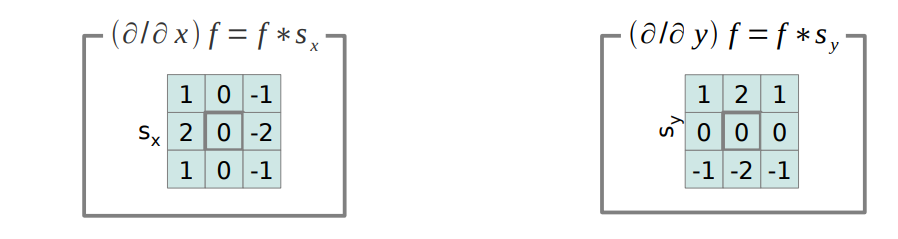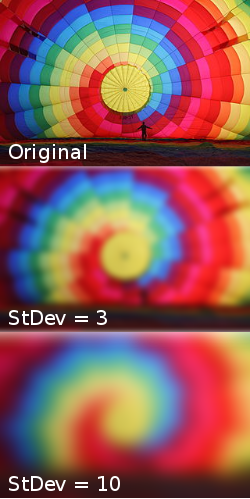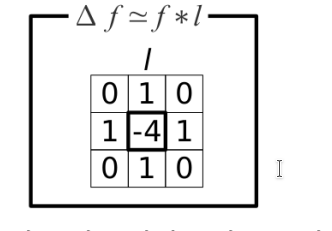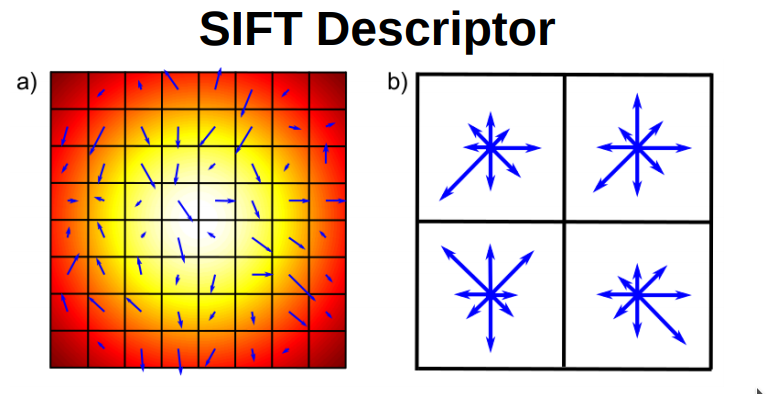# Overview of  a few convolution operators

### Sobel Operator for Edge DetectionThe Sobel Operator calculates the pixels first order derivatives in the X and Y direction.

If the is little derivative, there is no unstructured area

If the derivative high in one direction, we have an edge in that direction

If the derivative high in two directions  , we have a corner / point of interest### Gaussian Smoothing

Replace each pixel by it’s local average

.It is used for scale Space

### The Laplace  Operator forThe differnce compard to the Sobel operator is, that it uses the second order derrivative.

This makes the Laplace operator very sensitive to noisy.

Edges are, where the second derivative is crossing over to 0 ( Zweite ableitung = 0 Hochpunkt!)

2. ### Then find zero crossings with the Laplacian filter.

3. Can also combine one into one LOG  convolution

### Double of Gaussian

LoG does not have to be calculated, it can be also approximated by calculating the difference between two Gaussian Filters at different scales . DoG

### SIFT Detector

1. Multiple DoG filters are applied to the image at different scales
2. The resulting images are stacked on top each other to create a 3D volume
3. Points that are local extrema inside of the 3D voluma, are considerd points of interest.
4. Remove bad points, like candidates in smooth regions  or directly on top of edges

### SIFT Discriptor1. For a detected point of interest, choose 16×16 region around the point
2. Compute each gradient for each pixel
3. Subdivide into 16 * 4×4 groups
4. Compute orientation histogram
5. Glue the histograms together to get a 128 element feature vector

#### Characteristics

• SIFT is very resillient to changes (invariant)   to constant intensity changes, as itt is based on gradients
• Very invariant to contreast changes  , as the histogram binning provides normalization
• invarient to small deformations

Scale invariance is caused by the SIFT detector